# JavaScript Basics #1

JavaScript is the first programming language we are going to introduce in this tutorial. It was created in 1995 as a way to add programs to web pages in the Netscape Navigator browser. Today the language has been adopted by all the other major web browsers, and it has become one of the most popular programming languages in the world.

## Setting up the environment #

In this tutorial, we are going to have a lot of example code snippets. To execute the code, we can simply open a browser, go to Developer Tools -> Console: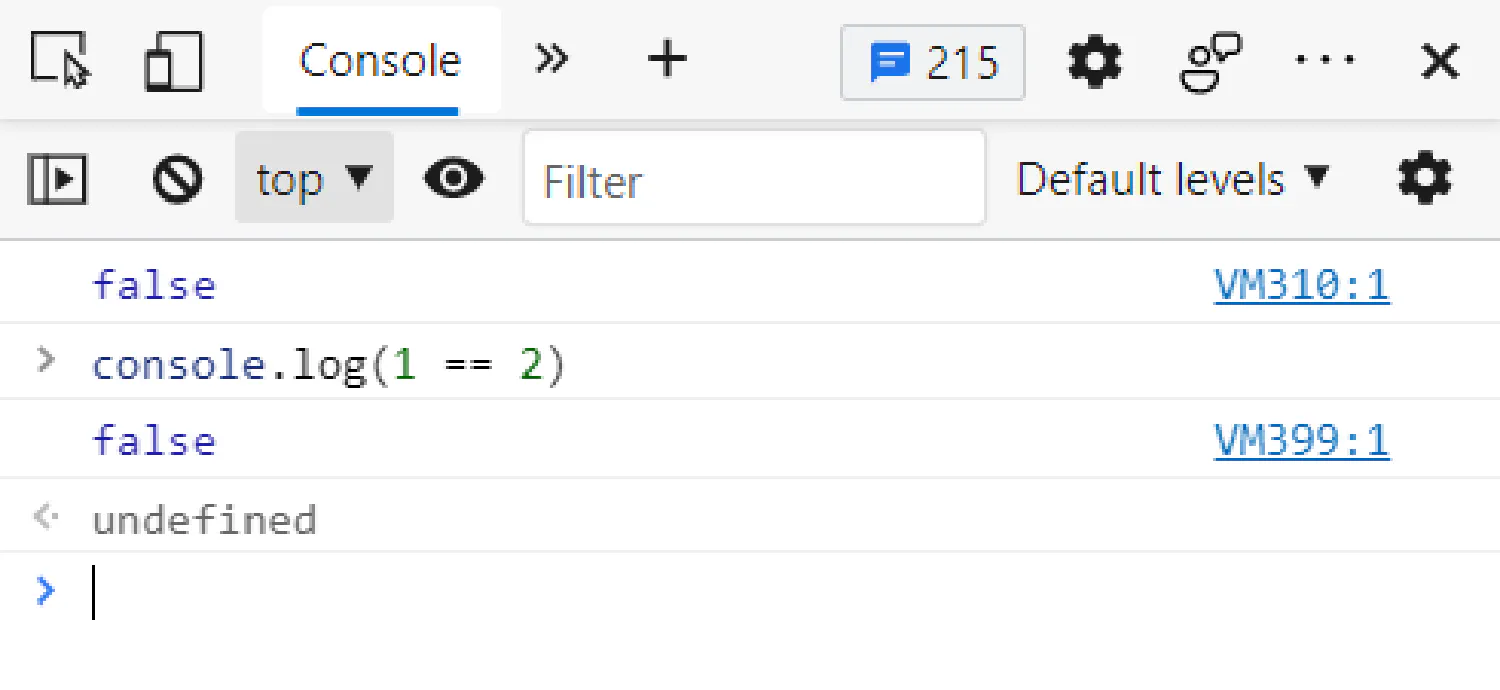Or you can install Node.js on your computer, which allows you to run JavaScript programs using command terminals.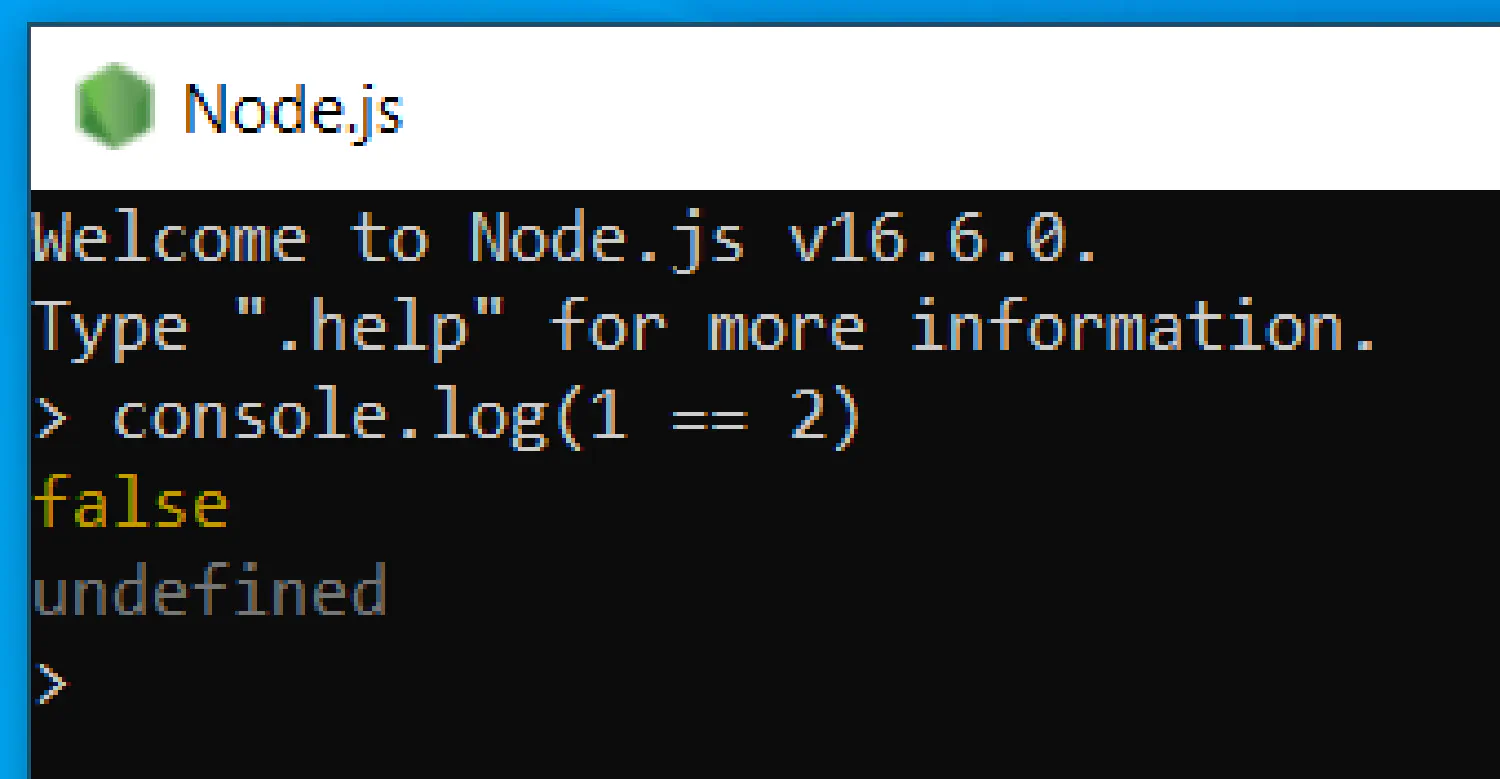## Data types in JavaScript #

In the computer world, it’s all about data. What a computer program does is essentially take some input data, process them, and then return some output data. In this section, let’s talk about some different types of data that JacaScript can process.

### Numbers #

Numbers are the easiest because it works exactly like what you studied in your math class in elementary school.

 ``````1 2 3 4 5 6 7 8 `````` ``````// Integer 100; // Fractional Number 10.56; //Scientific Notation 3.14e5; // 3.14 * 10^5 = 314000 ``````

The primary usage of numbers is to perform arithmetic operations.

 ``````1 `````` ``````3 + 5 * 2; // -> 13 ``````

Just like you studied in elementary school, multiplications and divisions happen first. However, you can change this by using parentheses.

 ``````1 `````` ``````(3 + 5) * 2; // -> 16 ``````

There is one operator that you might not recognize, which is the modulo (`%`) operation. `X % Y` calculates the remainder of dividing `X` by `Y`. For example:

 ``````1 2 3 4 5 `````` ``````25 % 5; // -> 0 25 % 10; // -> 5 25 % 15; // -> 10 ``````

### Strings #

Strings are used to represent texts, and they are all enclosed in quotes like this:

 ``````1 2 `````` ``````"This is a string."; "This is also a string."; ``````

Both single and double quotes work exactly the same, as long as the opening and the closing quotes match each other.

Whenever a backslash (`\`) is found inside a string, it means the character after it has a special meaning. For example, when the backslash is followed by the letter n (`\n`), this will be interpreted by your computer as a new line:

 ``````1 `````` ``````"This is the first line\nThis is the second line"; ``````

The output text would look like this:

 ``````1 2 `````` ``````This is the first line This is the second line ``````

The `+` operation can also be used on strings as well. But obviously, strings can not be used in arithmetic operations, the plus sign here means concatenate (connecting two strings together).

 ``````1 `````` ``````"con" + "cat" + "e" + "nate"; // -> "concatenate" ``````

Finally, there is a special kind of string in JavaScript, the backtick-quoted strings, usually called template literals. It allows us to embed other values inside the string:

 ``````1 `````` ```````half of 100 is \${100 / 2}`; ``````

In this example, the division inside `\${}` will be calculated, the result will be converted into a string and printed in that position. So this example will give us:

 ``````1 `````` ``````half of 100 is 50 ``````

### Boolean values #

The Boolean type only includes two values, `true` and `false`. Comparison is the most common way to produce boolean values.

 ``````1 2 3 4 5 6 7 `````` ``````console.log(1 == 1); // -> true console.log(1 > 2); // -> false console.log(1 < 0); // -> false console.log(1 != 2); // -> true ``````

In this example, `==` means equal, and `!=` means not equal. Other similar operators include `>=` (greater than or equal to) and `<=` (less than or equal to).

There are three logical operators that we can apply to Boolean values in JavaScript, `&&` (and), `||` (or), and `!` (not).

The `&&` operator denotes logical `and`, it produces `true` only if both values given to it are `true`.

 ``````1 2 3 4 5 6 7 `````` ``````console.log(true && false); // -> false console.log(false && true); // -> false console.log(false && false); // -> false console.log(true && true); // -> true ``````

The `||` operator denotes logical `or`, it produces `true` if either of the values given to it is `true`.

 ``````1 2 3 4 5 6 7 `````` ``````console.log(true || false); // -> true console.log(false || true); // -> true console.log(false || false); // -> false console.log(true || true); // -> true ``````

The `!` operator denotes logical `not`, and it flips the given value.

 ``````1 2 3 `````` ``````console.log(!true); // -> false console.log(!false); // -> true ``````

We can also mix arithmetic operations with comparisons and logical operations.

 ``````1 `````` ``````1 + 1 == 2 && 1 + 1 < 0; ``````

In this example, `1 + 1 == 2` gives us `true`, and `1 + 1 < 0` gives us `false`, so we have

 ``````1 `````` ``````true && false; // -> false ``````

### Empty values #

There are two special values in JavaScript, `null` and `undefined`. They indicate the absence of a meaningful value. In computer programs, there are a lot of operations that do not produce meaningful results (which we will see later in this course), and these results will be denoted by `null` or `undefined`.

These two values have virtually no difference, in fact, in most cases, you can treat them as interchangeable. The fact that there are two different values indicating the same thing is just an accident of JavaScript’s design.

### Data type conversion #

JavaScript is a very intelligent programming language, it will always try to execute the program you give it, even though the program does not make sense. For example:

 ``````1 2 3 4 5 `````` ``````console.log(8 * null); // -> 0 console.log("5" - 1); // -> 4 console.log("5" + 1); // -> "51" ``````

In the first example, the `null` gets converted into the number `0`, and in the second example, the string `"5"` becomes the number `5`. However, in the third example, the number `1` gets converted into the string `"1"`, and the plus sign here means concatenate, so the result becomes `"51"`.

I know these results are all over the place, and they don’t make sense at all. This is why you should never try to do this when you are coding, even though it “works”, it will lead to unexpected results.

## Program structures in JavaScript #

### Statements and bindings #

In computer programming, you can think of a “program” as an instruction manual to solve a complex problem. Each instruction/sentence in that manual is called a statement. In JavaScript, a statement should always end with a semicolon(`;`).

 ``````1 `````` ``````let num = 10; ``````

This example is called a binding, or variable. It binds the value `10` to the name `num` using the `=` operator, which allows us to do something like this:

 ``````1 2 `````` ``````let num = 10; console.log(num * num); // -> 100 ``````

The keyword `let` indicates that this statement is going to define a binding. When a binding is formed, it does not mean that the name is tied to the value forever, we can still use the `=` operator on existing bindings.

 ``````1 2 3 4 5 `````` ``````let num = 10; console.log(num); // -> 10 num = 20; console.log(num); // -> 20 ``````

Notice that we only used the keyword `let` in line 1. That is because `let` is only used to declare a binding, and in line 5, we are merely updating the value that is tied to the variable `num`.

 `````` 1 2 3 4 5 6 7 8 9 10 `````` ``````let num1 = 10; let num2 = 20; console.log(num1); // -> 10 console.log(num2); // -> 20 num2 = num1; console.log(num1); // -> 10 console.log(num2); // -> 10 ``````
 ``````1 2 3 4 `````` ``````let num = 10; num = num - 5; console.log(num); // -> 5 ``````

The keywords `const` and `var` can also be used to create bindings just like `let`, however, they are different in terms of scopes, which we will in detail later.

### Functions #

A function is a piece of program that returns a value or has some side effects, or both. For example, the `console.log()` function we have seen a few times is used to output values in the terminal.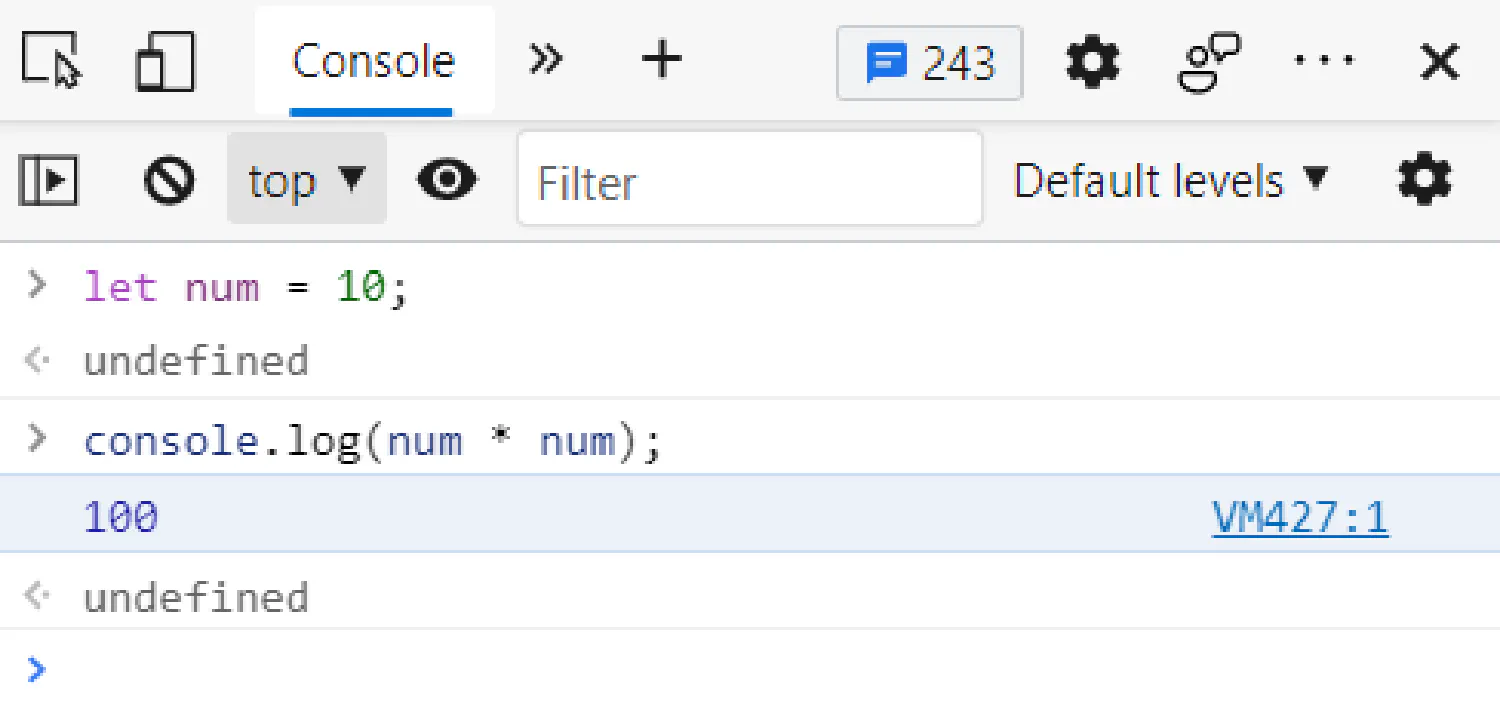Or, in this example, the `prompt()` function will show you a dialog that asks for user input, and that input will be bound with the variable `num`.

 ``````1 2 `````` ``````let num = prompt("Enter A Number"); console.log(num); ``````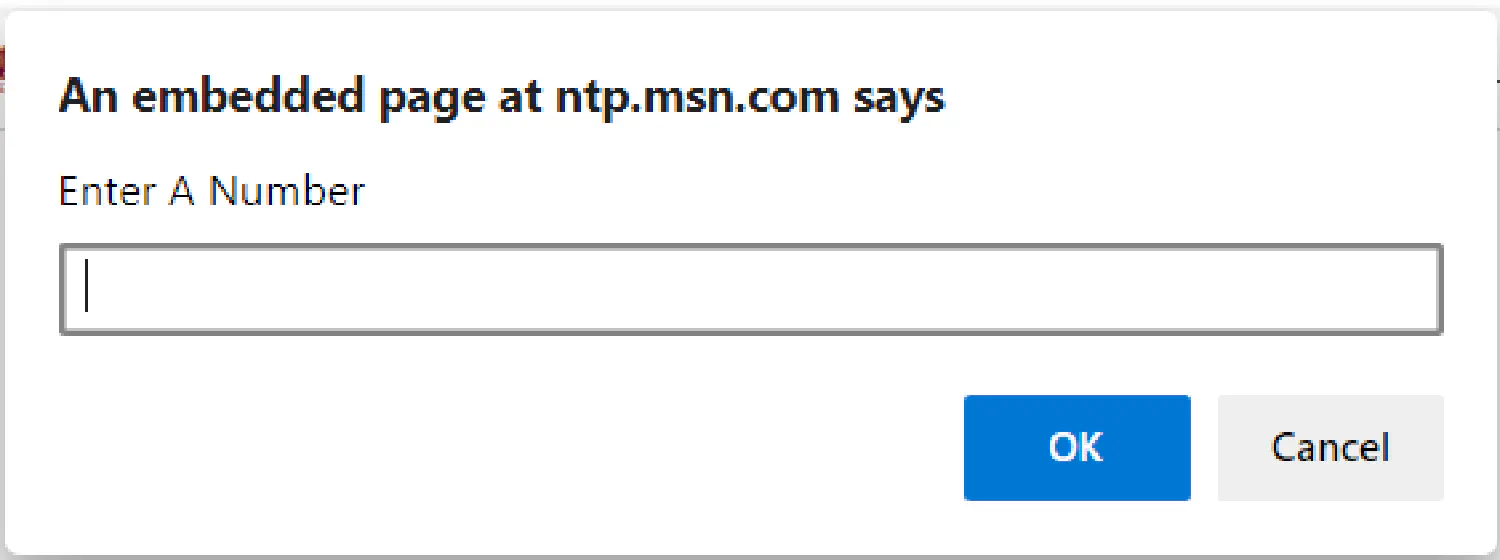Both showing a dialog and writing text to screen are side effects. A function can also be useful without the side effect. For example:

 ``````1 `````` ``````console.log(Math.max(2, 4, 6, 8)); ``````

The `Math.max()` function does not have any side effects, it simply takes a set of numbers and returns the greatest.

All of these functions are built into JavaScript. We can, however, create our own functions using JavaScript. We will discuss this topic in the next section.

### `if` statements #

The `if` statement offers us a way to execute different pieces of code under different conditions. For example:

 ``````1 2 3 4 5 6 7 `````` ``````let num = prompt("Enter A Number"); if (num < 10) { console.log("Small"); } else { console.log("Large"); } ``````

This program asks you to input a number, if the number is less than 10, `console.log("Small");` will be executed, and the program will output `"Small"`. If the number is larger than 10, the program will output `"Large"`.

We can also chain multiple `if`/`else` pairs if there are multiple conditions we need to consider:

 ``````1 2 3 4 5 6 7 `````` ``````if (num < 10) { console.log("Small"); } else if (num < 100) { console.log("Medium"); } else { console.log("Large"); } ``````

This program will first check if the number is less than 10, if it is, it will output `"Small"`. If the number is greater than 10, the program will then check if it is less than 100. If it is, the program will output `"Medium"`. Finally, if the number is greater than 100, the program will show `"Large"`.

### `for` loops #

The for loops offer us a way to execute the same code over and over again, as long as some conditions are satisfied.

 ``````1 2 3 `````` ``````for (let num = 0; num <= 12; num = num + 2) { console.log(num); } ``````

A `for` loop takes three expressions, separated by two semicolons. In this example, the first expression `let num = 0` declares a new variable `num`, whose initial value is 0. The second expression means the loop will iterate until the condition `num <= 12` is violated (`num` is larger than 12). The last expression means for each iteration, `num` will add itself by 2.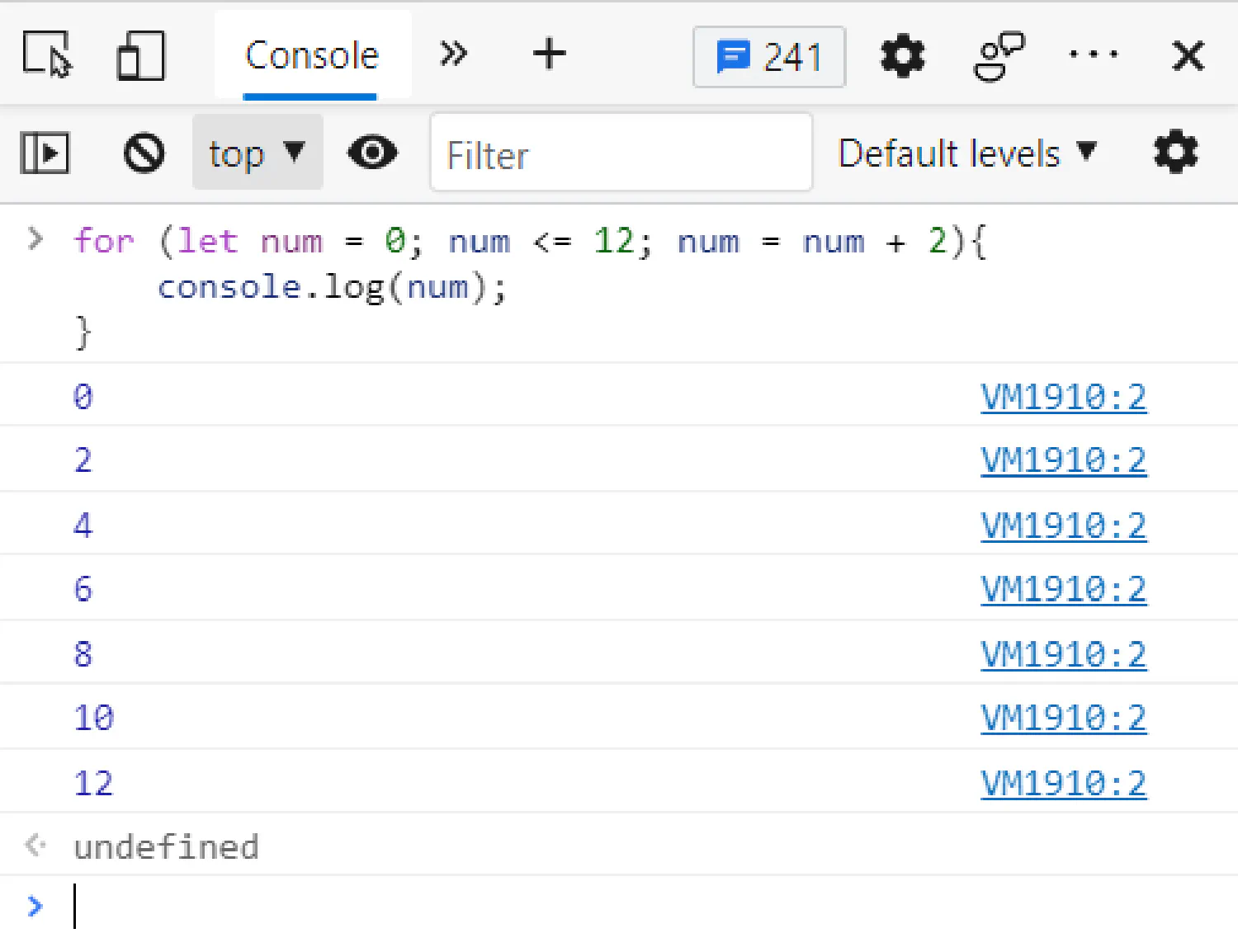### `while` loops #

`while` loops work in a similar way, except it only takes one expression. In fact, we can easily change our previous `for` loop example into a `while` loop.

 ``````1 2 3 4 5 `````` ``````let num = 0; while (num <= 12) { console.log(num); num = num + 2; } ``````

In this example, we initiated the `num` variable first, outside of the `while` loop. Inside the parentheses, after the keyword `while` is the expression that checks whether the loop should continue. Finally, we update the value of `num` at the end of the `while` loop.

### `do while` loops #

A `do-while` loop differs from a `while` loop only on one point, it guarantees that the body of the loop executes at least once.

 ``````1 2 3 4 5 `````` ``````let num = 10; do { num = num + 1; console.log(num); } while (num <= 1); ``````

This time the initial value of `num` is 10, which violates the condition for the loop to continue. But because this is a `do-while` loop, the body is still executed once. If this was a `while` loop, it would not execute at all.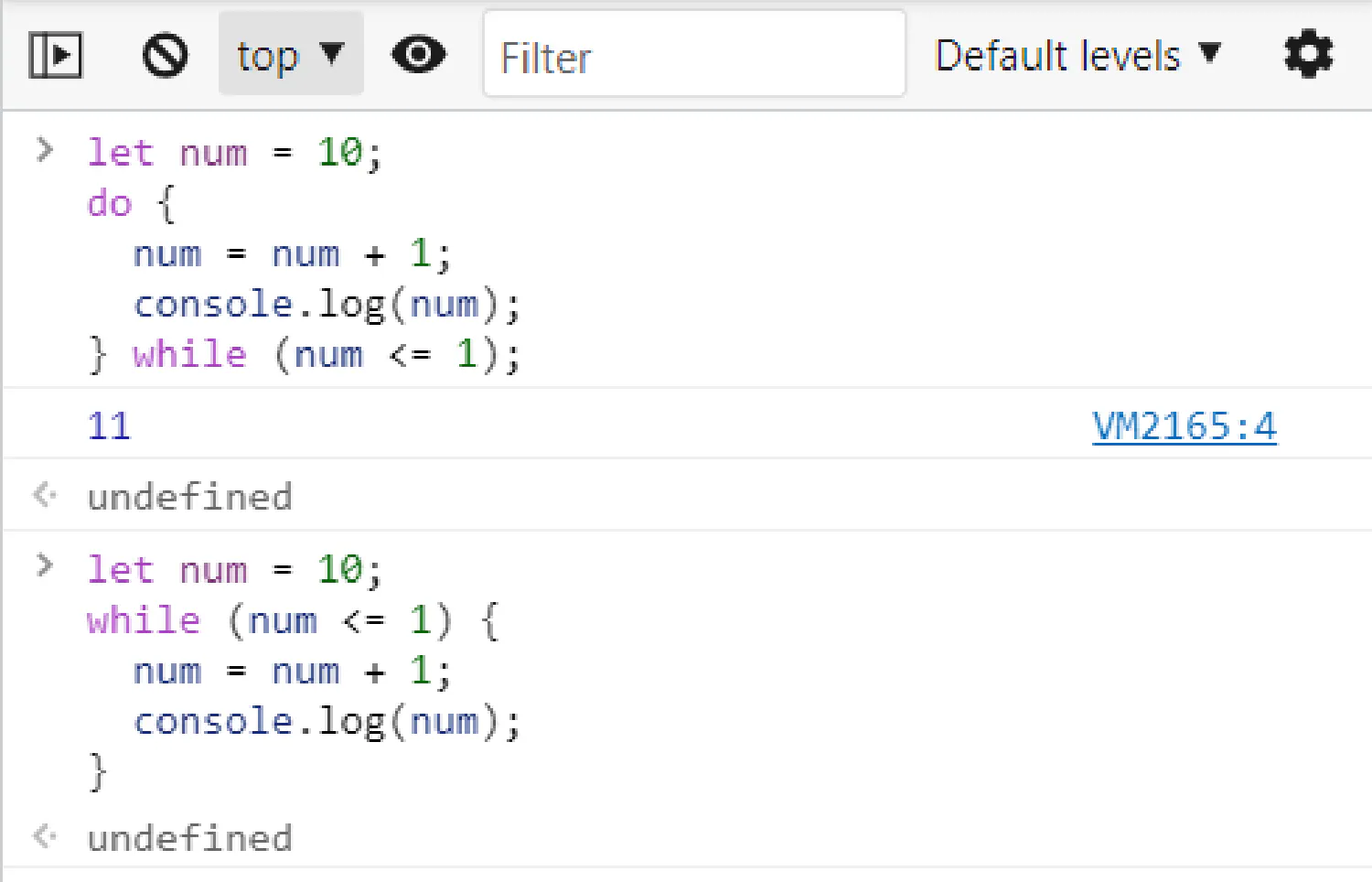### Breaking out of a loop #

Violating the condition for the loop to continue is not the only way we can stop a loop. For instance, you are asked to find a number that is greater than 100, and divisible by 9 (Recall that `%` operator is used to calculate reminder, so if the remainder of `x/9` equals 0, that means `x` is divisible by 9.). We can use a `for` loop to solve this problem:

 ``````1 2 3 4 5 6 `````` ``````for (let num = 100; ; num = num + 1) { if (num % 9 == 0) { console.log(num); break; } } ``````

Notice that we do not have an expression that decides whether the loop should continue. Instead, we have an `if` statement with a `break` keyword inside, which will break out of the loop if it is executed. If you remove the `break` keyword, this `for` loop becomes an infinite loop and will run forever, which is something you should always avoid.

## Functions in JavaScript #

Previously, we’ve seen some functions that come with JavaScript. In this section, we are going to focus on defining our own custom functions in JavaScript. A function can be seen as a piece of code wrapped in a value, which allows us to reuse that piece of code over and over again. In this article, we are going to talk about three different ways we can define a function in JavaScript.

The first method is to define functions as values, and bind that value to a name (like how we defined variables in the previous article).

 ``````1 2 3 `````` ``````let square = function (x) { return x * x; }; ``````

The function is created with the keyword `function`, and it will take a set of parameters as input, in this case, only `x`. A function should also have a body where you return an output using the keyword `return`, or have some kind of side effect. Lastly, the function as a value will be assigned to the name `square`, which we need to use to invoke this function.

Also, remember that the semicolon (`;`) at the end is necessary because it is still a full statement where you declare a binding, except the value here is a function.

 ``````1 `````` ``````console.log(square(10)); // -> 100 ``````

A function can have more than one parameter or no parameters at all (an empty set of parameters).

 ``````1 2 3 `````` ``````const sleep = function () { console.log("zzzzzzzzzzzzzzzzzzzzzz"); }; ``````
 ``````1 2 3 `````` ``````var multiply3 = function (x, y, z) { return x * y * z; }; ``````

As you can see, it is possible for a function to have only a side effect and not return anything.

The second method is slightly shorter, by declaring a function using the `function` keyword, and it doesn’t require a semicolon at the end:

 ``````1 2 3 `````` ``````function square(x) { return x * x; } ``````

The method also allows us to do something like this:

 `````` 1 2 3 4 5 6 7 8 9 10 `````` ``````sleep(); multiply3(2, 3, 4); function sleep() { console.log("zzzzzzzzzzzzzzzzzzzzzz"); } function multiply3(x, y, z) { return x * y * z; } ``````

Here we put the function declarations after the statement that calls them, and the code still works. Now, we can put all the functions in one place, which is a good thing for future maintenance.

The third method is called arrow functions. Instead of the keyword `function`, we can use an arrow (`=>`) to declare a function.

 ``````1 2 3 `````` ``````const square = (x) => { return x * x; }; ``````

This is the exact same `square()` function we saw before, and it works exactly the same. Then why does JavaScript have both arrow functions and the `function` keyword? While, in some cases, it allows us to write shorter functions.

If the function only has one parameter, then you can omit the parentheses around the parameter list. And if there is only one statement in the function body, the curly braces and the `return` keyword can also be omitted. Then, our `square()` function becomes:

 ``````1 `````` ``````const square = (x) => x * x; ``````

### Bindings and scopes #

Before we go deeper into the topic of functions, let’s go back to the first method. You may have noticed that we defined the functions in the examples using different keywords, `let`, `const` and `var`. What exactly are their differences?

First, we need to understand the concept of scope. It is the part of the program in which the binding is accessible. If a binding is defined outside of any functions or blocks (blocks can be `if` statements, `for` or `while` loops, etc.), then you can refer to that binding wherever you want. This is called a global binding.

If the binding is declared inside a function or block using `let` or `const`, that binding will only be accessible from inside the function/block, and that is called a local binding. However, if the binding is defined using the keyword `var`, then that binding will also be accessible from outside of the function/block.

 ``````1 2 3 4 5 6 7 8 9 `````` ``````let x = 10; if (true) { let y = 20; var z = 30; console.log(x + y + z); // -> all three variables are accessible here } console.log(x + z); // -> you cannot "see" y from here, but z is still accessible ``````

Now, what are the differences between `let` and `const`? As the name suggests, `const` stands for constant, meaning once a binding is declared using `const`, you cannot change its value (unlike `let`).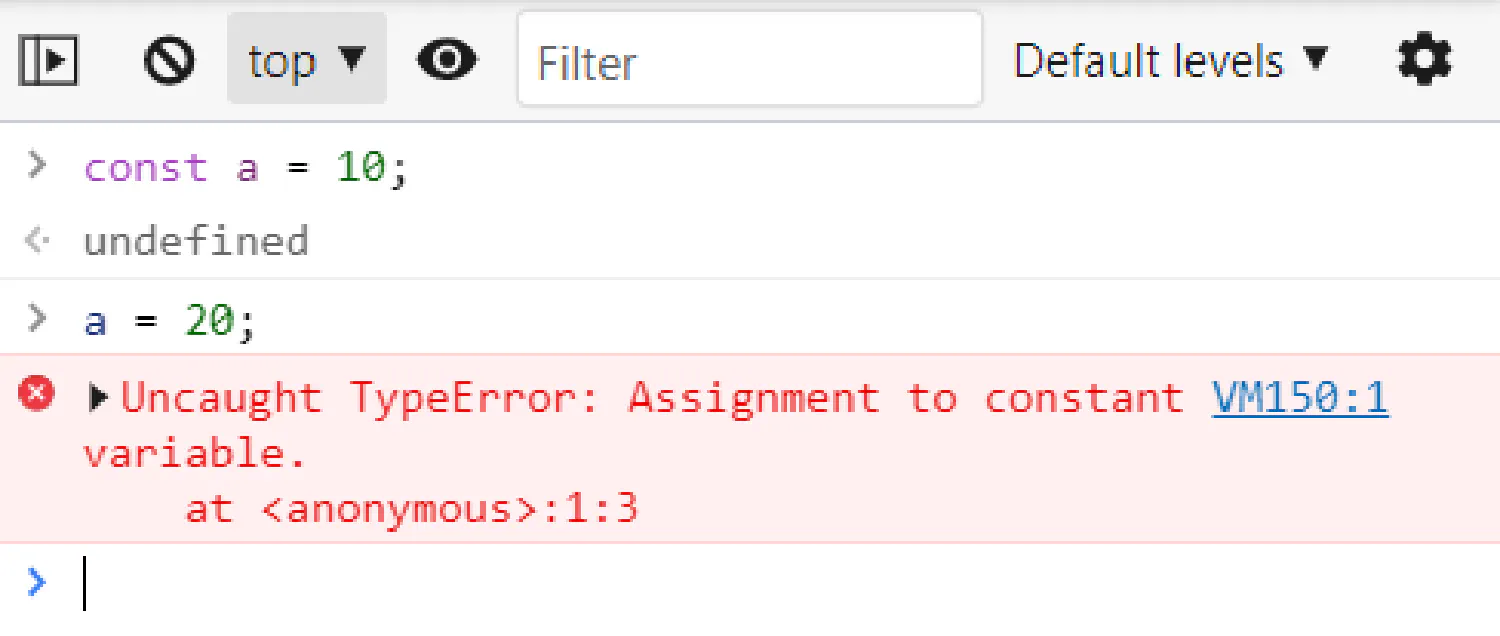### Optional arguments #

JavaScript is very broad-minded when it comes to the number of parameters you pass to the function. For example, we have the `square()` function we defined before, which is supposed to take one argument.

 ``````1 2 3 4 `````` ``````function square(x) { return x * x; } console.log(square(4, true, "qwerty")); ``````

In this example, we gave the `square()` function more than one argument, and it simply ignores the extra arguments and computes the square of the first one.

And if we passed too few arguments, those missing parameters will be assigned the value `undefined` instead of giving you an error.

The downside of this is, of course, when you accidentally make a mistake, no one will tell you about it. So, even though it technically works, you should never rely on this, it could give you some unexpected results. Instead, you should always be careful how many parameters you need, and how many arguments you are passing to the function.

### Rest parameters #

However, what if you don’t know how many parameters you need? For example, you are designing a function that finds the maximum number in a series of numbers, but you don’t know how many numbers are in the series, so you need to design a function that takes any number of arguments.

To write a function like this, you need to put three dots before the function’s last parameter:

 `````` 1 2 3 4 5 6 7 8 9 10 11 `````` ``````function max(...numbers) { let result = -Infinity; for (let number of numbers) { if (number > result) { result = number; } } return result; } max(1, 2, 3, 4, 5, 6, 7); ``````

Now, the parameter `numbers` (it is called the rest parameter) will be bound to an array, and the function will return the maximum number in that array.

An array is a list of items, in this case, we have `[ 1, 2, 3, 4, 5, 6, 7 ]`, and `for (let number of numbers)` is how we can iterate over all items in this array. We’ll discuss arrays in the next article.

## Recursions in JavaScript #

Finally, let’s talk about the concept of recursion. Recursion is when a function calls itself. The most typical example is how we calculate the power of a number.

 ``````1 2 3 4 5 6 7 `````` ``````function power(base, exponent) { if (exponent == 0) { return 1; } else { return base * power(base, exponent - 1); } } ``````

Notice that in line 5, the function `power()` called itself with parameters `base` and `exponent - 1`. I know this is a bit confusing, but don’t worry, to understand this code, let’s plug in some numbers. Let’s try to calculate `10^5` (10 to the power of 5).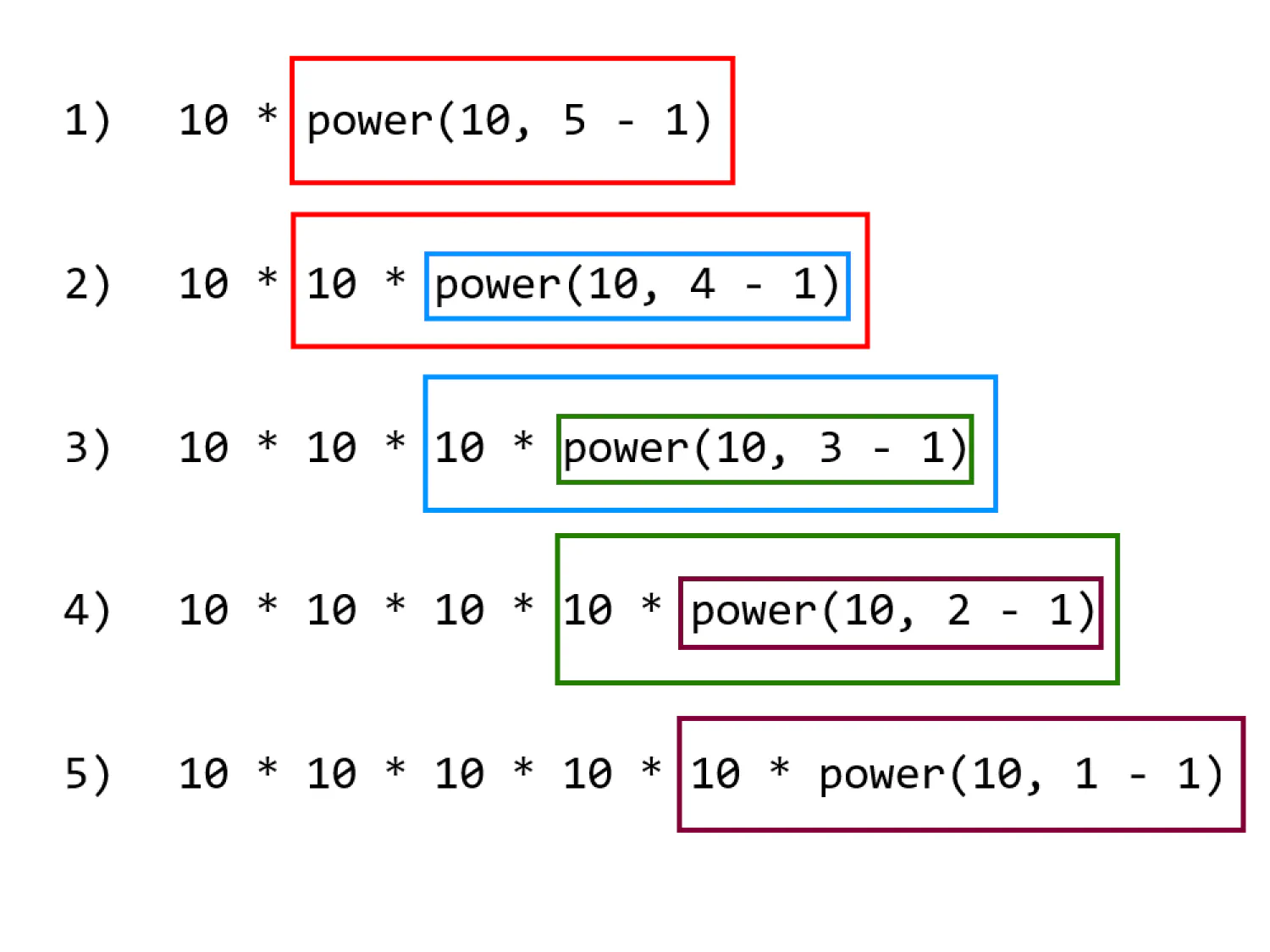In the first step, we simply plug in the numbers, and the function returns `10 * power(10, 4)`. Then we need to calculate `power(10, 4)`. Plug in the numbers, and we get `10 * power(10, 3)`, which means `power(10, 5)` equals `10 * 10 * power(10, 3)`.

And we keep repeating the same steps until we get `10 * 10 * 10 * 10 * 10 * power(10, 0)`. Because `power(10, 0)` returns `1`, eventually we get `power(10, 5)` equals `10 * 10 * 10 * 10 * 10`.

This is a very elegant way of defining exponentiation, but unfortunately, this method is about three times slower than using loops in JavaScript. This is a dilemma that programmers face all the time, we have to choose between simplicity and speed because almost any program can be made faster by making it bigger. It’s up to the programmer to decide on an appropriate balance.

If you think my articles are helpful to you, please consider making a donation to me. Your help is greatly appreciated.

## Subscribe to my newsletter ➡️

✅ News and tutorials every other Monday

✅ Unsubscribe anytime

✅ No spam. Always free.